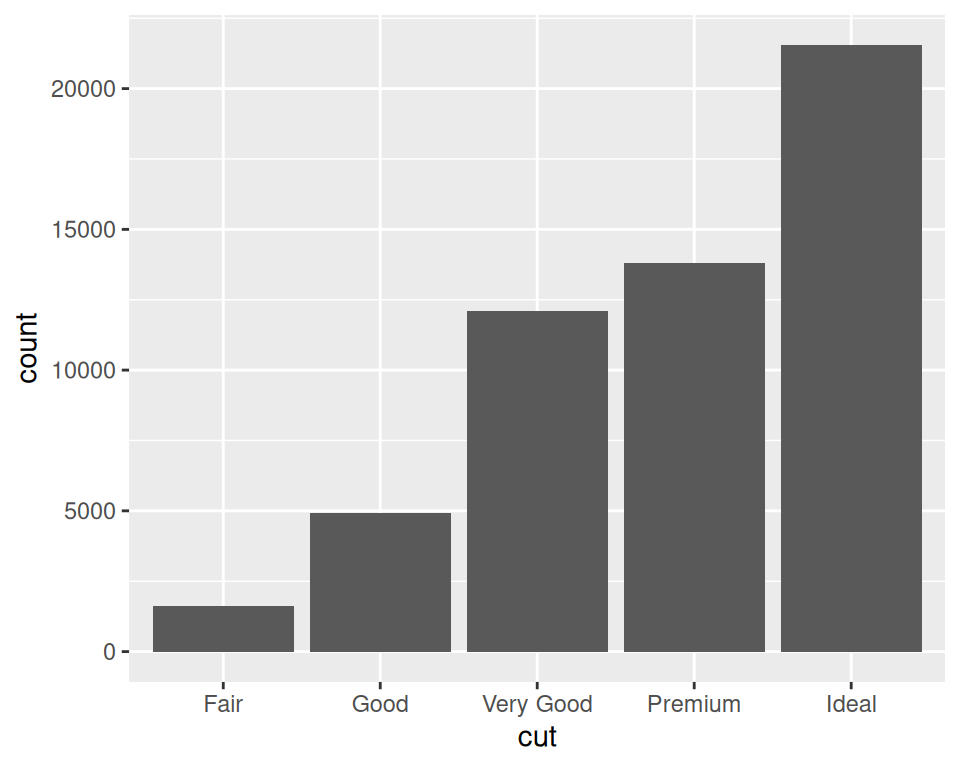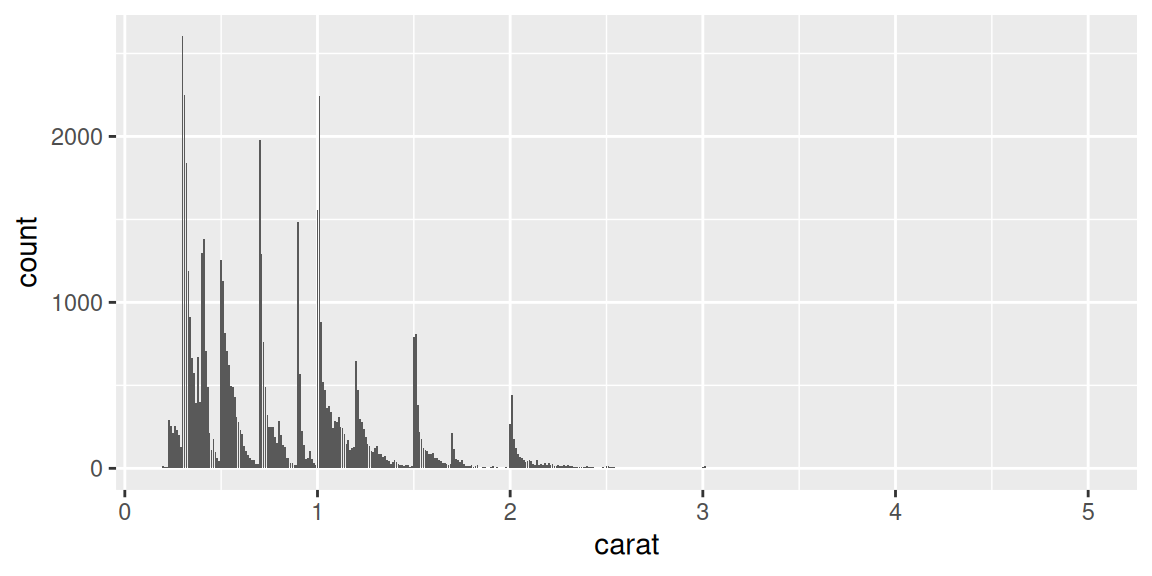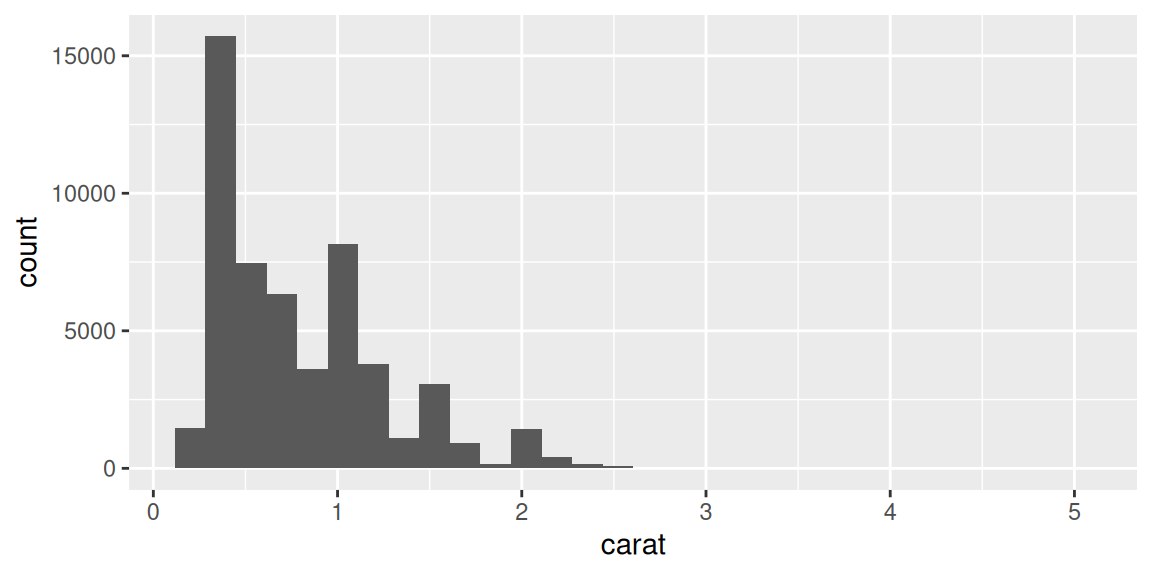## 3.3 Making a Bar Graph of Counts

### 3.3.1 Problem

Your data has one row representing each case, and you want plot counts of the cases.

### 3.3.2 Solution

Use `geom_bar()` without mapping anything to `y` (Figure 3.7):

``````# Equivalent to using geom_bar(stat = "bin")
ggplot(diamonds, aes(x = cut)) +
geom_bar()``````Figure 3.7: Bar graph of counts

### 3.3.3 Discussion

The `diamonds` data set has 53,940 rows, each of which represents information about a single diamond:

``````diamonds
#> # A tibble: 53,940 × 10
#>   carat cut       color clarity depth table price     x     y     z
#>   <dbl> <ord>     <ord> <ord>   <dbl> <dbl> <int> <dbl> <dbl> <dbl>
#> 1  0.23 Ideal     E     SI2      61.5    55   326  3.95  3.98  2.43
#> 2  0.21 Premium   E     SI1      59.8    61   326  3.89  3.84  2.31
#> 3  0.23 Good      E     VS1      56.9    65   327  4.05  4.07  2.31
#> 4  0.29 Premium   I     VS2      62.4    58   334  4.2   4.23  2.63
#> 5  0.31 Good      J     SI2      63.3    58   335  4.34  4.35  2.75
#> 6  0.24 Very Good J     VVS2     62.8    57   336  3.94  3.96  2.48
#> # ℹ 53,934 more rows``````

With `geom_bar()`, the default behavior is to use `stat = "bin"`, which counts up the number of cases for each group (each x position, in this example). In the graph we can see that there are about 23,000 cases with an `ideal` cut.

In this example, the variable on the x-axis is discrete. If we use a continuous variable on the x-axis, we’ll get a bar at each unique x value in the data, as shown in Figure 3.8, left:Figure 3.8: Bar graph of counts on a continuous axis (left); A histogram (right)

The bar graph with a continuous x-axis is similar to a histogram, but not the same. A histogram is shown on the right of Figure 3.8. In this kind of bar graph, each bar represents a unique x value, whereas in a histogram, each bar represents a range of x values.

If, instead of having `ggplot()` count up the number of rows in each group, you have a column in your data frame representing the y values, use `geom_col()`. See Recipe 3.1.
You could also get the same graphical output by calculating the counts before sending the data to `ggplot()`. See Recipe 15.17 for more on summarizing data.